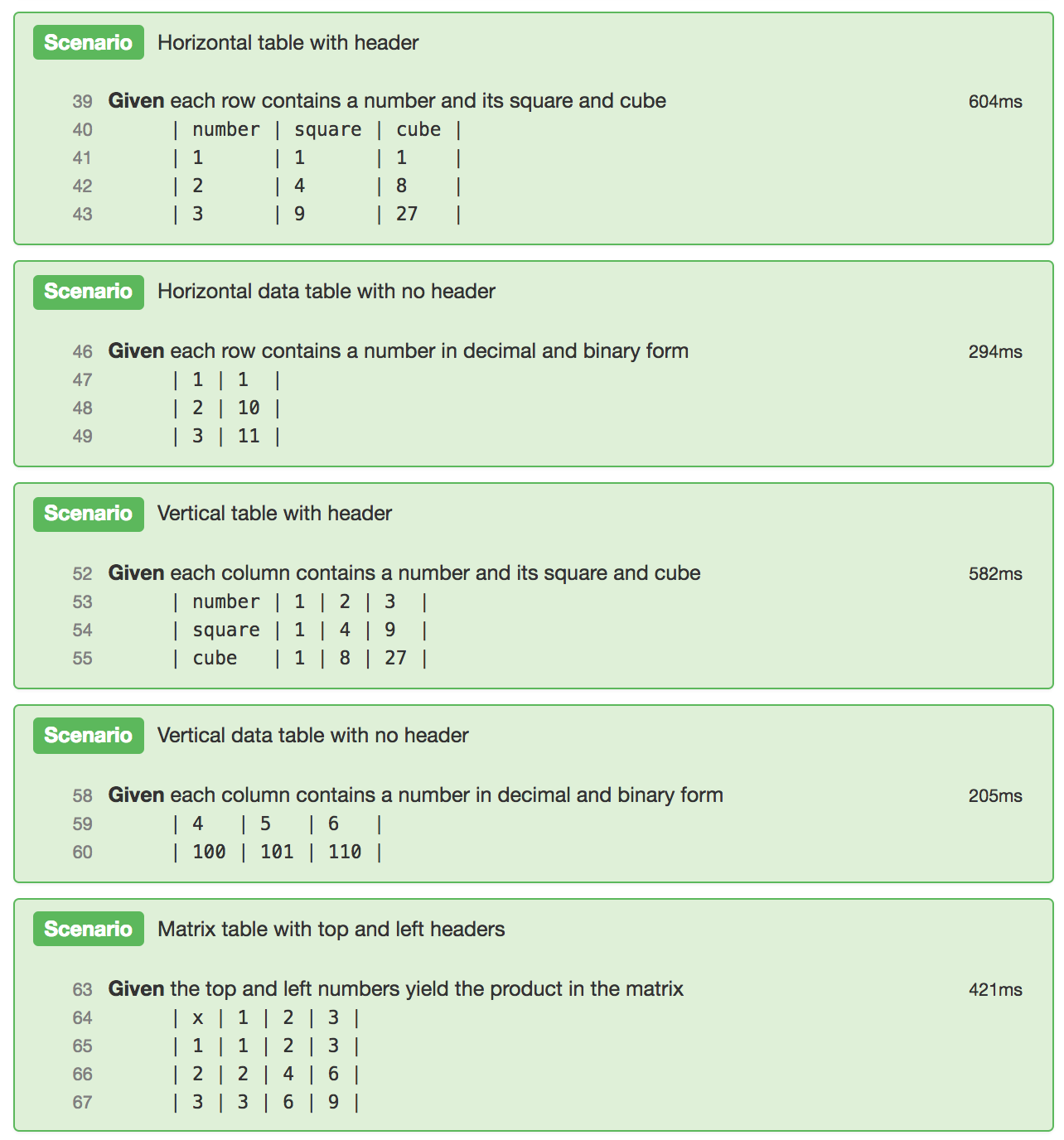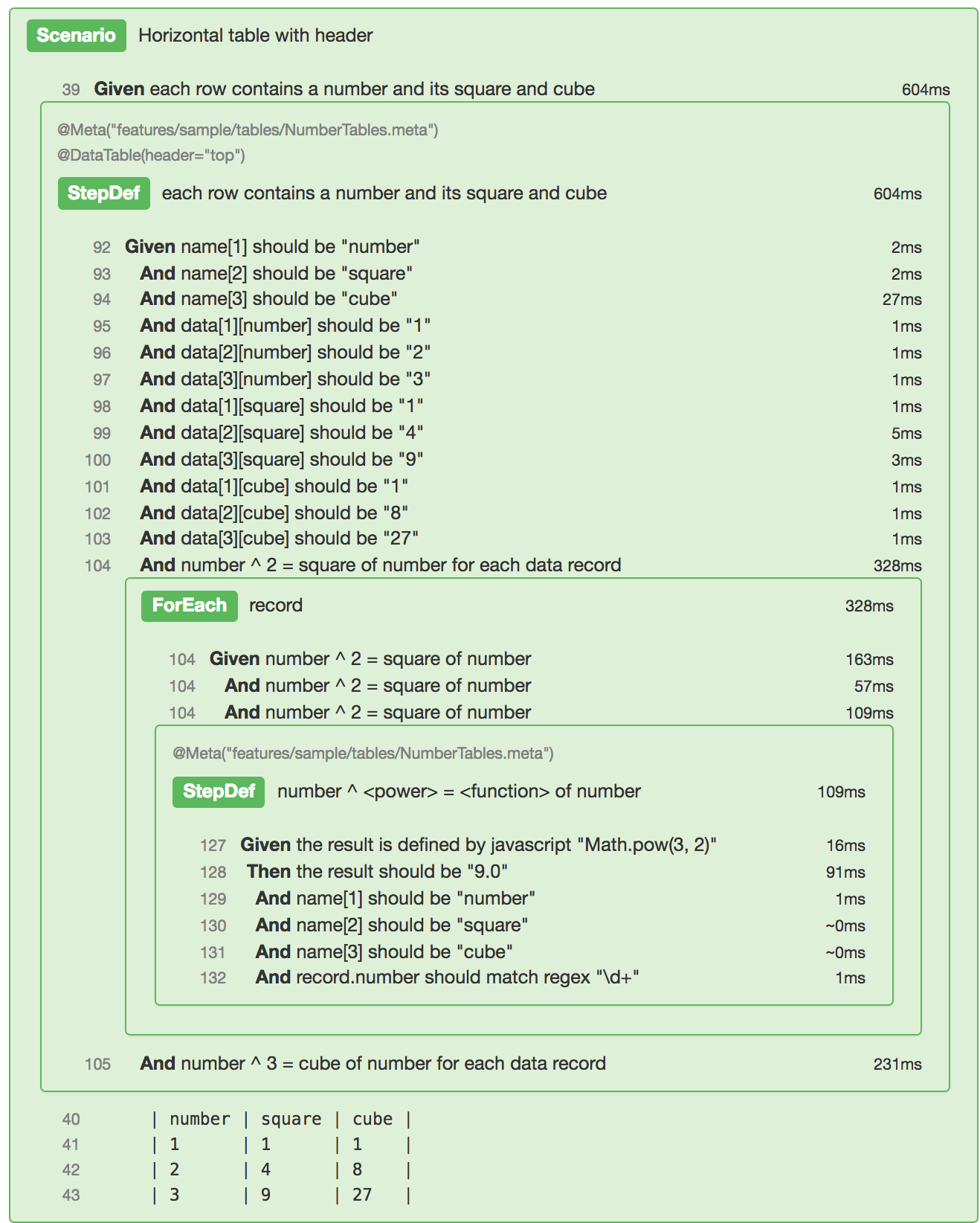# Data Tables# Data Tables

If a step contains a data table, then a `@StepDef` scenario must be declared for it and be annotated with a `@DataTable` tag to make the contained data accessible as shown in the examples below.

# Horizontal Data Tables

This example has 3 rows and 3 columns of data and a top header. The first horizontal row is the header and the rows that follow are data records.

### In feature..

```   Scenario: Horizontal table with header
Given each row contains a number and its square and cube
| number | square | cube |
| 1      | 1      | 1    |
| 2      | 4      | 8    |
| 3      | 9      | 27   |```

### In meta..

We specify the `@DataTable` tag on the StepDef with the `header` attribute set to `top` to assign the names in the top header record to the elements in each data record. We then reference the data using `data[recordNo][name]` to access any explicit record (where `recordNo` of 1st record is 1, 2nd is 2, and so on), or `data[name]` to access the current record in a 'for each' step.

```  @StepDef
Scenario: each row contains a number and its square and cube
Given name should be "number"
And name should be "square"
And name should be "cube"
And data[number] should be "1"
And data[number] should be "2"
And data[number] should be "3"
And data[square] should be "1"
And data[square] should be "4"
And data[square] should be "9"
And data[cube] should be "1"
And data[cube] should be "8"
And data[cube] should be "27"
And number ^ 2 = square of number for each data record
And number ^ 3 = cube of number for each data record

@StepDef
Scenario: number ^ <power> = <function> of number
Given the result is defined by javascript "Math.pow(\${data[number]}, \$<power>)"
Then the result should be "\${data[\$<function>]}.0"```

This example has 3 rows and 2 columns of data and no header. Each horizontal row is a data record.

### In feature..

```   Scenario: Horizontal data table with no header
Given each row contains a number in decimal and binary form
| 1 | 1  |
| 2 | 10 |
| 3 | 11 |```

### In meta..

We specify the `@DataTable` tag on the StepDef with the `horizontal` attribute set to `decimal,binary` to assign names to the elements in each data record. We then reference the data using `data[recordNo][name]` to access any explicit record (where `recordNo` of 1st record is 1, 2nd is 2, and so on), or `data[name]` to access the current record in a 'for each' step.

```  @StepDef
@DataTable(horizontal="decimal,binary")
Scenario: each row contains a number in decimal and binary form
Given name should be "decimal"
And name should be "binary"
And data[decimal] should be "1"
And data[decimal] should be "2"
And data[decimal] should be "3"
And data[binary] should be "1"
And data[binary] should be "10"
And data[binary] should be "11"
And the decimal data[decimal] should be data[binary] in binary for each data record

@StepDef
Scenario: the decimal <decimal> should be <binary> in binary
Given the result is defined by javascript "Number(\${\$<decimal>}).toString(2)"
Then the result should be "\${\$<binary>}"```

# Vertical Data Tables

This example has 3 rows and 3 columns of data and a left header. The first vertical column is the header and the columns that follow are data records.

### In feature..

```   Scenario: Vertical table with header
Given each column contains a number and its square and cube
| number | 1 | 2 | 3  |
| square | 1 | 4 | 9  |
| cube   | 1 | 8 | 27 |```

### In meta..

We specify the `@DataTable` tag on the StepDef with the `header` attribute set to `left` to assign the names in the left header record to the elements in each data record. We then reference the data using `data[recordNo][name]` to access any explicit record (where `recordNo` of 1st record is 1, 2nd is 2, and so on), or `data[name]` to access the current record in a 'for each' step.

```  @StepDef
Scenario: each column contains a number and its square and cube
Given name should be "number"
And name should be "square"
And name should be "cube"
And data[number] should be "1"
And data[number] should be "2"
And data[number] should be "3"
And data[square] should be "1"
And data[square] should be "4"
And data[square] should be "9"
And data[cube] should be "1"
And data[cube] should be "8"
And data[cube] should be "27"
And number ^ 2 = square of number for each data record
And number ^ 3 = cube of number for each data record
@StepDef
Scenario: number ^ <power> = <function> of number
Given the result is defined by javascript "Math.pow(\${data[number]}, \$<power>)"
Then the result should be "\${data[\$<function>]}.0"```

This example has 2 rows and 3 columns of data and no header. Each vertical row is a data record.

### In feature..

```   Scenario: Vertical data table with no header
Given each column contains a number in decimal and binary form
| 4   | 5    | 6   |
| 100 | 101  | 110 |```

### In meta..

We specify the `@DataTable` tag on the StepDef with the `vertical` attribute set to `decimal,binary` to assign names to the elements in each data record. We then reference the data using `data[recordNo][name]` to access any explicit record (where `recordNo` of 1st record is 1, 2nd is 2, and so on), or `data[name]` to access the current record in a 'for each' step).

```  @StepDef
@DataTable(vertical="decimal,binary")
Scenario: each column contains a number in decimal and binary form
Given name should be "decimal"
And name should be "binary"
And data[decimal] should be "4"
And data[decimal] should be "5"
And data[decimal] should be "6"
And data[binary] should be "100"
And data[binary] should be "101"
And data[binary] should be "110"
And the decimal data[decimal] should be data[binary] in binary for each data record

@StepDef
Scenario: the decimal <decimal> should be <binary> in binary
Given the result is defined by javascript "Number(\${\$<decimal>}).toString(2)"
Then the result should be "\${\$<binary>}"```

# Matrix Tables

A matrix has a top and left headers around contained data. That data can only be accessed by top-left coordinates and so there is no concept of records in a matrix.

This example has 3 rows and 3 columns of data and top and left headers. The first row (excluding the first element is the top header and the first column (excluding the first element) is the left header. The data exists below and to the right of these headers respectively.

### In feature..

```   Scenario: Matrix table with top and left headers
Given the top and left numbers yield the product in the matrix
| x | 1 | 2 | 3 |
| 1 | 1 | 2 | 3 |
| 2 | 2 | 4 | 6 |
| 3 | 3 | 6 | 9 |```

### In meta..

We specify the `@DataTable` tag on the StepDef with the `type` attribute set to `matrix` to assign top-left header coordinates to the data elements in the matrix. We can then reference the names in the top and left headers using `top.name[columnNo]` and `left.name[rowNo]` respectively (where both `columnNo` and `rowNo` are 1 for the 1st header element, 2 for 2nd and so on). The name of the header element at top-left corner can be accessed as `vertex.name`. The data can be accessed using `data[top-name][left-name]` (where `top-name` and `left-name` are the top and left header names of the row and column where the data resides - they just happen to also be numbers in this example).

```  @StepDef
@DataTable(type="matrix")
Scenario: the top and left numbers yield the product in the matrix
Given vertex.name should be "x"
And top.name should be "1"
And top.name should be "2"
And top.name should be "3"
And left.name should be "1"
And left.name should be "2"
And left.name should be "3"
And data should be "1"
And data should be "2"
And data should be "3"
And data should be "2"
And data should be "4"
And data should be "6"
And data should be "3"
And data should be "6"
And data should be "9"
And top.name x left.name = data
And top.name x left.name = data
And top.name x left.name = data
And top.name x left.name = data
And top.name x left.name = data
And top.name x left.name = data
And top.name x left.name = data
And top.name x left.name = data
And top.name x left.name = data

@StepDef
Scenario: <a> x <b> = <c>
Given the product is defined by javascript "\${\$<a>} * \${\$<b>}"
Then the product should be "\${\$<c>}"```

## Implicit table data attributes

The following attributes are implicitly available to data records processed by the for each:

• `record.number` = The record number starting at 1
• `record.index` = The record index starting at 0 (available since gwen 2.18.2 and gwen-web 2.30.1)

## Sample Reports

### Compact### Expanded##### Clone this wiki locally
You can’t perform that action at this time.